Previously we discussed that both GAAP and IRS uses different scheduling techniques for depreciation. For the ease of understanding we assumed the depreciation rate to be equivalent to the cost-of capital rate. But now for real life complications we need to understand how to tackle this difference in scheduling techniques and how to reverse engineer the firm reports and what the firm actually pays.

A disclosed financial statement might contain what the GAAP requires but the IRS depreciation schedule might not match with the disclosed statements. For example say you released a financial statement stating \$25 depreciation for 3 years. But in reality the IRS might allow for a more accelerated form of depreciation which can be \$60 in the first followed by \$15 in the next year.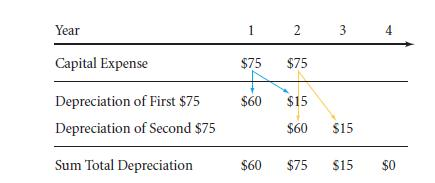You might argue that both the representations are logically same in financial terms, but if you take a look at the disclosed financial figures for the IRS department, you will find they are not the same with the undisclosed figures.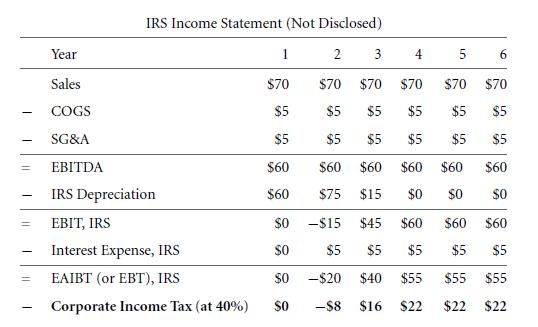A quick comparison between the GAAP determined income tax table and the real IRS financial table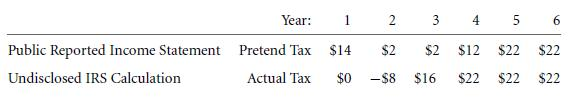Both the tables show a sum total of \$74. But the total might be equal, but a closer look tells you that the figures for the first two years in public disclosed statement are higher than real and lower in the last two years. The reason behind this is that the IRS allowed a quicker depreciation than GAAP allowed.

If you wish to work with the real IRS financials then you will be disappointed to know that no corporate companies disclose their true IRS statements. But the good news is that you will get your hands on the difference between the real taxes and the GAAP permitted taxes. These figures are known as deferred taxes and are calculated from the balance sheet. For a better understanding we assume a hypothetical number that will provide the amount by which your company has over reported their taxes on the financial statement: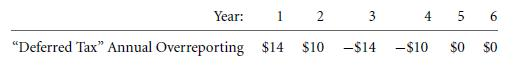You are required to report the cumulative sum with the above amount:You will find that in the balance sheet the above deferred tax figure is reported under liability section. This can be better viewed as an amount by which you have understated your real income by overstating your real income tax. In order to calculate the real income tax figure from the fake reported income tax you need to put to use your reverse engineering skills. The procedure is explained in the following:

1. Find the overstated deferred tax value per year. This can be done by finding every consecutive annual increment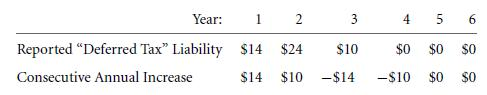1. Find the actual paid tax amount by subtracting the above numbers from public disclosed tax statements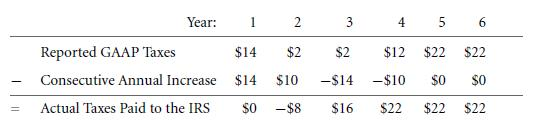1. To get the original financial figures before tax payment subtract the original tax numbersIf you wish to find out the financial statements involving after tax payment cash flows you need to do either of the following

• Add back the GAAP taxes which were subtracted and then subtract real IRS taxes.
• Add back the increase in deferred tax

Example: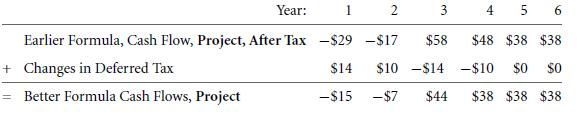To sum up what we learned till now abut extracting cash flows from finance statements: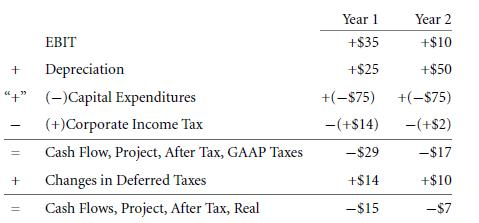With that we come to the conclusion of our discussion about difference between GAAP and IRS.

Links of Next Financial Accounting Topics:-### Customer Reviews

My Homework Help
Rated 5.0 out of 5 based on 510 customer reviews at